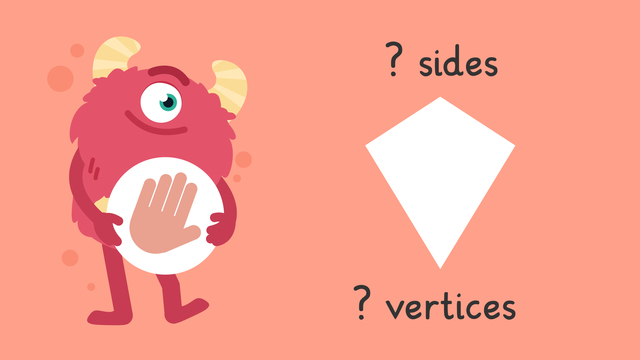# Sides and Vertices of 2-D Shapes — Let's Practice!Rating

Ø 3.0 / 2 ratings
The authorsTeam Digital
Sides and Vertices of 2-D Shapes — Let's Practice!
CCSS.MATH.CONTENT.4.G.A.2

## Basics on the topicSides and Vertices of 2-D Shapes — Let's Practice!

Today we are practicing identifying sides and vertices of 2-D shapes with Razzi! This video contains examples to help you further practice and grow confident in this area.

### TranscriptSides and Vertices of 2-D Shapes — Let's Practice!

Razzi says get these items ready because today we're going to practice sides and vertices of 2D Shapes It's time to begin! Let's find how many sides and vertices some 2D shapes have! Look at this shape. How many sides does it have? How many vertices? Did you also get four sides and four vertices? Let's tackle the next problem! Look at this shape. How many sides does it have? How many vertices? Did you also get eight sides and eight vertices? Let's tackle the next problem! Look at this irregular shape. How many sides does it have? How many vertices? Did you also get ten sides and ten vertices? Let's tackle the final problem! Look at this irregular shape. How many sides does it have? How many vertices? Did you also get seven sides and seven vertices? Razzi had so much fun practicing with you today! See you next time!

## Sides and Vertices of 2-D Shapes — Let's Practice! exercise

Would you like to apply the knowledge you’ve learned? You can review and practice it with the tasks for the video Sides and Vertices of 2-D Shapes — Let's Practice! .
• ### How many sides and vertices?

Hints

The sides are the straight lines all around the outside of the 2D shape. Count the sides. This shape has four sides.

The vertices are the point where two sides meet. Here they are circled. How many can you see?

Solution

This shape is a pentagon. It has 5 sides and 5 vertices.

• ### Features of a hexagon.

Hints

The sides are the straight lines all around the edge of the shape. How many can you count?

This image shows the sides and vertices of a pentagon. This pentagon has 5 sides and vertices. Does the hexagon have more or fewer?

Solution

This shape is a hexagon. It has 6 sides and 6 vertices.

• ### How many sides?

Hints

The sides are the straight lines all around the edge of the shape. How many can you count?

This shape is a trapezoid. It has 4 sides.

Solution
• The orange shape is a triangle and has 3 sides.
• The yellow shape is a star and has 10 sides.
• The purple shape is a hexagon and has 6 sides.
• The red shape is a hexagon and has 6 sides.

Hints

Count the straight lines around the edge of the shape, this is the number of sides.

A circle is a special kind of shape, because it has no straight sides.

Solution
• The first shape is an irregular pentagon, it has 5 sides and 5 vertices.
• The second shape is a circle, it has 0 sides and 0 vertices.
• The third shape is a regular pentagon, it has 5 sides and 5 vertices.
• ### Sides and vertices of a rectangle.

Hints

This square shows one vertex and one side labelled.

The vertices are where two sides meet, there are four vertices on a square.

Solution

The rectangle has four sides and four vertices. Here is one side and one vertex labelled.

• ### What am I?

Hints

What is special about a circle?

A shape with 5 sides is called a pentagon.

Solution
• What am I? I am a 2D shape with 5 vertices and 5 sides. I am a pentagon.
• What am I? I am a 2D shape with 6 vertices and 6 sides. I am a hexagon.
• What am I? I am a 2D shape but I have no vertices and no sides. I am a circle!